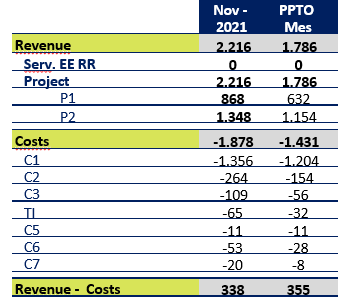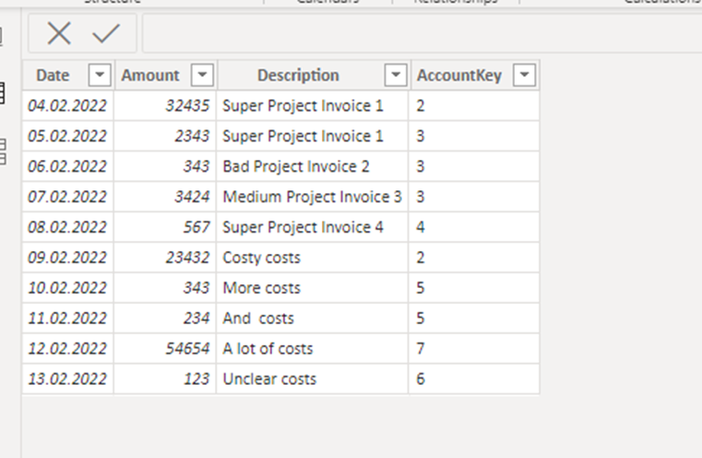cancel
Showing results for
Did you mean:Frequent Visitor

## Add row with result of calculation

Hello to all ! I am new to the world of Power Bi. There are things that I still can't figure out if it is possible or how it could be possible.
I currently have the following problem:

I have a table that displays information,
but I need to be able to have a row or a table that shows the result between the operation revenue - Costs (for example, because I have a line that takes out the tax, among other financial calculations that are performed today in excel) , as seen in this image :Is it possible to have such a revenue - cost line?

Or is it possible to have a separate table with this information? For example, something like this:1 ACCEPTED SOLUTIONHelper IV

Hi,

basically there is no simple solution to your problem. You must build up some data structure do this. This is all described in the “DAX Patterns” book, chapter 18 “Parent-child hierarchies”.

I will give the explanation with a simpler toy model. You will need an account hierarchy table and the actual bookings/postings for the cost and revenue itemsYou define input table the account id, the account name, the account type (expense or income), the operator (+/-) and the parent key

• Parent key: defines the summation of every child to the parent
• Operator: defines the summation sign. Here all costs are summed up and all revenue and then the difference is taken.

If you think about it , this is always a tree if you draw all parent child relationships as graph.

Now there are a few calculated columns:

AccountPath =

PATH ( Account[AccountKey], Account[ParentKey] )

Level1 =

VAR LevelNumber = 1

VAR LevelKey =

PATHITEM ( Account[AccountPath], LevelNumber, INTEGER )

VAR LevelName =

LOOKUPVALUE ( Account[AccountName], Account[AccountKey], LevelKey )

VAR Result = LevelName

RETURN

Result

This traverses the tree to get the name of ancestor of the level n.

The other 2 Levels look the same formula-wise. This is a crucial point; you know the levels of your tree in advance and define all accordingly.

Depth =

PATHLENGTH ( Account[AccountPath] )

This just gives you the depth of the item.

The actual costs are in a booking/postings table. With the above structure you only must assign your booking to an account number.Then you have to establish one to many relationship between the accounts and the postings via the AccountKey

Now you need a few measures:

Sum Amount =

SUM ( Postings[Amount] )

Total =

VAR Val =

SUMX (

SUMMARIZE ( Postings, Account[AccountType] ),

VAR SignToUse =

IF ( Account[AccountType] IN { "Expense" }, -1, +1 )

VAR Amount = [Sum Amount]

RETURN

Amount * SignToUse

)

VAR AccountShowRow = [AccountBrowseDepth] <= [AccountRowDepth]

VAR Result =

IF ( AccountShowRow, Val )

RETURN

Result

AccountRowDepth =

MAX ( Account[Depth] )

AccountBrowseDepth =

ISINSCOPE ( Account[Level1] ) + ISINSCOPE ( Account[Level2] )

+ ISINSCOPE ( Account[Level3] )

This is the part where you have to understand what you want: there is some account in the tree and all the descendants should be summed into this item (this all bookings with an account that is a descendant of this account).The above is the toy model result: You  have the levels in the row of the matrix which defines the specific filter context. If you take “Income” for example, this is level 2 and all level 3 items in the tree that are children of “Income” are summed up.

The measure works as follows:

• Via SUMMARIZE every booking is identified either with expense or income. This is necessary to define the sign
• The amount is then the sum of all bookings in the filter context which is according to the level.
• The AccountShowRow variable makes sure that only  bookings for children are and bookings for the item itself are summed

This seems a lot of work, for what you asked. On the other hand you have to set up the account structure only once (or it least it is slowly changing).
Then your bookings must be assigned to accounts, and this is then all.

ToyModel.pbix

Best regards

ChristianHelper IV

Hi,

basically there is no simple solution to your problem. You must build up some data structure do this. This is all described in the “DAX Patterns” book, chapter 18 “Parent-child hierarchies”.

I will give the explanation with a simpler toy model. You will need an account hierarchy table and the actual bookings/postings for the cost and revenue itemsYou define input table the account id, the account name, the account type (expense or income), the operator (+/-) and the parent key

• Parent key: defines the summation of every child to the parent
• Operator: defines the summation sign. Here all costs are summed up and all revenue and then the difference is taken.

If you think about it , this is always a tree if you draw all parent child relationships as graph.

Now there are a few calculated columns:

AccountPath =

PATH ( Account[AccountKey], Account[ParentKey] )

Level1 =

VAR LevelNumber = 1

VAR LevelKey =

PATHITEM ( Account[AccountPath], LevelNumber, INTEGER )

VAR LevelName =

LOOKUPVALUE ( Account[AccountName], Account[AccountKey], LevelKey )

VAR Result = LevelName

RETURN

Result

This traverses the tree to get the name of ancestor of the level n.

The other 2 Levels look the same formula-wise. This is a crucial point; you know the levels of your tree in advance and define all accordingly.

Depth =

PATHLENGTH ( Account[AccountPath] )

This just gives you the depth of the item.

The actual costs are in a booking/postings table. With the above structure you only must assign your booking to an account number.Then you have to establish one to many relationship between the accounts and the postings via the AccountKey

Now you need a few measures:

Sum Amount =

SUM ( Postings[Amount] )

Total =

VAR Val =

SUMX (

SUMMARIZE ( Postings, Account[AccountType] ),

VAR SignToUse =

IF ( Account[AccountType] IN { "Expense" }, -1, +1 )

VAR Amount = [Sum Amount]

RETURN

Amount * SignToUse

)

VAR AccountShowRow = [AccountBrowseDepth] <= [AccountRowDepth]

VAR Result =

IF ( AccountShowRow, Val )

RETURN

Result

AccountRowDepth =

MAX ( Account[Depth] )

AccountBrowseDepth =

ISINSCOPE ( Account[Level1] ) + ISINSCOPE ( Account[Level2] )

+ ISINSCOPE ( Account[Level3] )

This is the part where you have to understand what you want: there is some account in the tree and all the descendants should be summed into this item (this all bookings with an account that is a descendant of this account).The above is the toy model result: You  have the levels in the row of the matrix which defines the specific filter context. If you take “Income” for example, this is level 2 and all level 3 items in the tree that are children of “Income” are summed up.

The measure works as follows:

• Via SUMMARIZE every booking is identified either with expense or income. This is necessary to define the sign
• The amount is then the sum of all bookings in the filter context which is according to the level.
• The AccountShowRow variable makes sure that only  bookings for children are and bookings for the item itself are summed

This seems a lot of work, for what you asked. On the other hand you have to set up the account structure only once (or it least it is slowly changing).
Then your bookings must be assigned to accounts, and this is then all.

ToyModel.pbix

Best regards

ChristianAnnouncements#### Exclusive opportunity for Women!

Join us for a free, hands-on Microsoft workshop led by women trainers for women where you will learn how to build a Dashboard in a Day!#### Power Platform Conference-Power BI and Fabric Sessions

Join us Oct 1 - 6 in Las Vegas for the Microsoft Power Platform Conference.Top Solution Authors
Top Kudoed Authors
Users online (3,511)